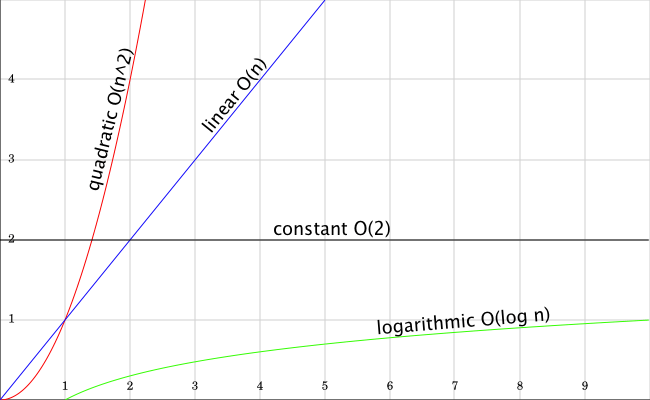# Leaena

## Time Complexity in Algorithms

Nov
29

Time complexity is a useful concept in programming. It’s essential for job interviews, but beyond that to really be a good programmer you need to know how cumbersome your algorithm is going to be. For 10 items, something that takes 2 seconds per item is only 20 seconds, but what if you have a million items?

In general, time complexity is measured by how long it takes a number of items (n) to be processed. It is the relationship between the number of things you have to do and the work to be done. Something that takes the same amount of time no matter have much of it you have to do, would be constant time. If I had 10 items or 1 million it would only take me 2 seconds to process. On another extreme is something called the handshake problem. If you only have two people in a room they only have to shake one another’s hands (one handshake). If you have 10 people, they each shake 9 other hands (55 handshakes total, we’ll get to the math in a moment). This is quadratic time.

Here’s a visual of the common programming time complexities:Lets start with constant time again. In programming, constant time is like deciding whether a number is even or odd:

`` n % 2 = ? ``

This is just one expression. You run and you’re done. It’s always going to take a certain amount of time, no matter what n you give it. In big O notation we would write this as O(1).

Next, my favorite, as far as understanding goes. Linear time. In programming, linear time, is like running through a loop of n numbers and doing something.

`````` for( var i = 0; i < n; i++ ){
do something..
}``````

The more things (‘n’s) you have, the longer the algorithm is going to take. It’s a 1:1 ratio. If 1 thing takes 1 second, 2 things are going to take 2 seconds. This is O(n).

Quadratic time is worse than linear time. It’s like the handshake problem or in programming terms a for loop within a for loop. For each n we need to run through all the numbers 1 through n.

`````` for( var i = 0; i < n; i++ ){
for( var j = 0; j < n; j++ ){
do something..
}
}``````

Each n thing is done n times. This is n * n or in big O terms O(n2).

Logarithmic time is all kinds of magic. It tapers off to a near constant for larger ‘n’s. This is possible because of the magic of halves. In a binary search tree there are only two options to descend, left (options lower than the current value) or right (options higher than the current value). Either choice will cut off roughly half of all the possible values. On the next level, you have the same choice, either you’ve found your value, or you go left or right, halving your options again.

`````` while ( low <= high ) {
var mid = ( low + high ) / 2;
if ( target < list[mid] ){
var high = mid - 1;
} else if ( target > list[mid] ){
var low = mid + 1;
} else { break; }
}``````

If you’re constantly halving the amount of things you have to check, eventually you hit a roughly constant time for an n search. This is O(log n).

This post has been brought to you by a forum post on time complexity, bits and pieces of the wiki article on time complexity, and this inspirational code class ad featuring my lead instructor from his Twitter days.

### 3 Responses to Time Complexity in Algorithms

1.Hey Lindsey, wouldn’t the handshakes between 10 people make it 54 hand shakes?

•Oops, we’re both off. It’s actually 45.

2.You are right and thanks for the link.